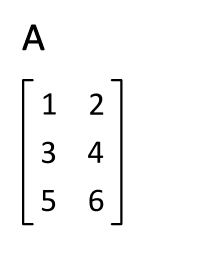16
Jun 18

## Matrix transposition: continuing learning by doing

Definition. For any matrix$A,$ its transposed$A^T$ is obtained by putting rows of$A$ into columns of$A^T$.Figure 1. Matrix transpose

Exercise 1. See Figure 1 borrowed from Wikipedia. Based on that illustration, what is the relationship between$A$ and$(A^T)^T?$

Exercise 2. For the matrix$A=\left(\begin{array}{cc}1&2\\3&4\\5&6 \end{array}\right)$ which of the products$AA^T$ and$A^TA$ exists? Find the one(s) that exist. Repeat the same for$A=\left(\begin{array}{c}1\\3\\5\end{array}\right).$

Definition. If$A=A^T,$ then$A$ is called symmetric.

Exercise 3. Can a non-square matrix be symmetric? The above definition is in matrix terms. What does it mean in terms of matrix elements?

Exercise 4. What is the relationship between$\det A$ and$\det (A^T)?$ Consider just a$2\times 2$ matrix.

Exercise 5. What happens if you apply transposition to a product$AB$?

SolutionPartitioning$A$  and$B$ into rows and columns, respectively, we find the elements of the product$C$ as dot products of the rows of$A$ by the columns of$B$:

(1)$C=\left(\begin{array}{ccc}A_1\cdot B^1&...&A_1\cdot B^k \\...&...&... \\A_n\cdot B^1&...&A_n\cdot B^k\end{array}\right)=\left(\begin{array}{ccc}A_1B^1&...&A_1B^k \\...&...&... \\ A_nB^1&...&A_nB^k\end{array}\right).$

In the second expression the dots are omitted because the$A_j$ are rows and the$B^i$ are columns, so that the matrix product definition can be applied to write$A_j\cdot B^i=A_jB^i.$ Further,$(B^i)^T$ are rows and$(A_j)^T$ are columns, so we can write

(2)$A_jB^i=(B^i)^T(A_j)^T.$

Transposing (1) and using (2) we have$C^T=\left(\begin{array}{ccc}A_1B^1&...&A_nB^1 \\...&...&... \\A_1B^k&...&A_nB^k\end{array} \right)=\left(\begin{array}{ccc}(B^1)^T(A_1)^T&...&(B^1)^T(A_n)^T \\...&...&... \\(B^k)^T(A_1)^T&...&(B^k)^T(A_n)^T\end{array}\right)$$=\left(\begin{array}{c}(B_1)^T \\... \\(B_k)^T\end{array}\right)\left(\begin{array}{ccc}(A^1)^T&...&(A^n)^T\end{array}\right)=B^TA^T.$

We have proved that$(AB)^T=B^TA^T.$

Exercise 6. What is the relationship between transposition and inversion? More precisely, if$A$ is invertible, then what can you say about$(A^T)^{-1}$?

Solution. By definition,$AA^{-1}=I.$ Transposing this gives$(A^{-1})^TA^T=I^T=I.$ This shows that$(A^T)^{-1}=(A^{-1})^T.$